# Getting started

• visualizing datasets in two or three dimensions;

• generating feature vectors for supervised learning;

• computing classical embeddings, like PCA and spectral embedding;

• drawing graphs in 2 or 3 dimensions;

Note

To learn how to create custom embeddings (with custom objective functions and constraints), sanity check embeddings, identify possible outliers in the original data, embed new data, and more, see the MDE guide. (We recommend reading the getting started guide first.)

## What is an embedding?

An embedding of a finite set of items (such as biological cells, images, words, nodes in a graph, or any other abstract object) is an assignment of each item to a vector of fixed length; the original items are embedded or mapped into a real vector space. The length of the vectors is called the embedding dimension. An embedding is represented concretely by a matrix, in which each row is the embedding vector of an item.

Embeddings provide concrete numerical representations of abstract items, for use in downstream computational tasks. For example, when the embedding dimension is 2 or 3, embeddings can be used to create a sort of chart or atlas of the items. In such a chart, each point corresponds to an item, and its coordinates in space are given by the embedding vector. These visualizations can help scientists and analysts identify patterns or anomalies in the original data, and more generally make it easier to explore large collections of data. PyMDE can embed into 2 or 3 dimensions, but it can also be used to embed into many more dimensions, which is useful when generating features for machine learning tasks.

For an embedding to be useful, it must be faithful to the original data (the items) in some way. To make it easy to get started, PyMDE provides two high-level functions for creating embeddings, based on related but different notions of faithfulness. These functions handle the common case in which each item is associated with either an original high-dimensional vector or a node in a graph. The functions are

The first creates embeddings that focus on the local structure of the data, putting similar items near each other and dissimilar items not near each other. The second focuses more on the global structure, choosing embedding vectors to respect some notion of original distance or dissimilarity between items.

We’ll see how to use these functions below.

## Visualizing data

When the embedding dimension is 2 (or 3), embeddings can be used to visualize large collections of items. These visualizations can sometimes lead to new insights into the data.

### Preserving neighbors

Let’s create an embedding that preserves the local structure of some data, using the `pymde.preserve_neighbors` function. This function is based on on preserving the `k`-nearest neighbors of each original vector (where `k` is a parameter that by default is chosen on your behalf).

We’ll use the MNIST dataset, which contains images of handwritten digits, as an example. The original items are the images, and each item (image) is represented by an original vector containing the pixel values.

```import pymde

mnist = pymde.datasets.MNIST()
```

Next, we embed.

```mde = pymde.preserve_neighbors(mnist.data, verbose=True)
embedding = mde.embed(verbose=True)
```

The first argument to `preserve_neighbors` is the data matrix: there are 70,000 images, each represented by a vector of length 784 , so `mnist.data` is a `torch.Tensor` of shape `(70,000, 784)`. The optional keyword argument `verbose=True` flag turns on helpful messages about what the function is doing. The embedding dimension is 2 by default.

The function returns a `pymde.MDE` object, which can be thought of as describing the kind of embedding we would like. To compute the embedding, we call the `embed` method of the `mde` object. This returns a `torch.Tensor` of shape `(70,000, 2)`, in which `embedding[k]` is the embedding vector assigned to the image `mnist.data[k]`.

We can visualize the embedding with a scatter plot. In the scatter plot, we’ll color each point by the digit represented by the underlying image.

```pymde.plot(embedding, color_by=mnist.attributes['digits'])
```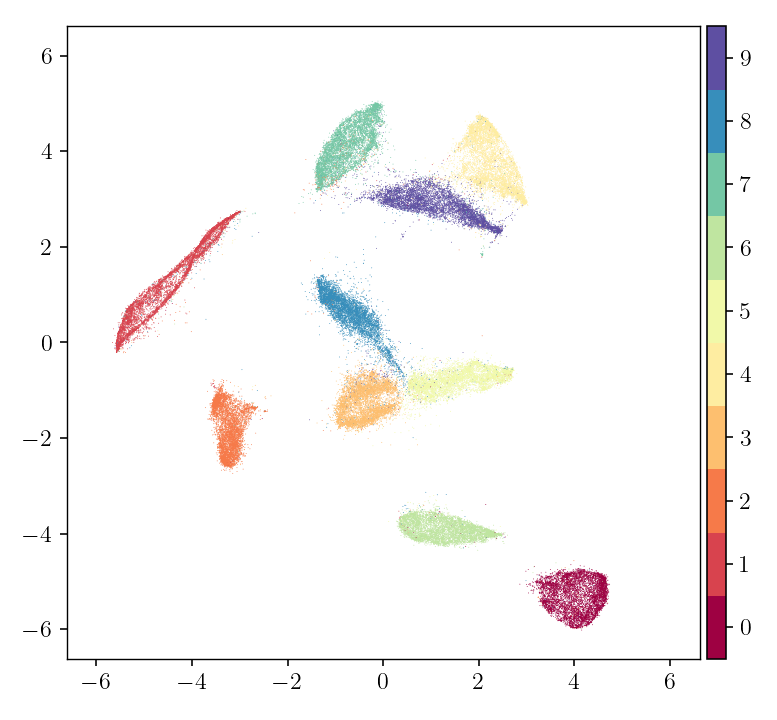We can see that similar images are near each other in the embedding, while dissimilar images are not.

It is also possible to embed into three or more dimensions. Here is an example with three dimensions.

```mde = pymde.preserve_neighbors(mnist.data, embedding_dim=3, verbose=True)
embedding = mde.embed(verbose=True)
pymde.plot(embedding, color_by=mnist.attributes['digits'])
```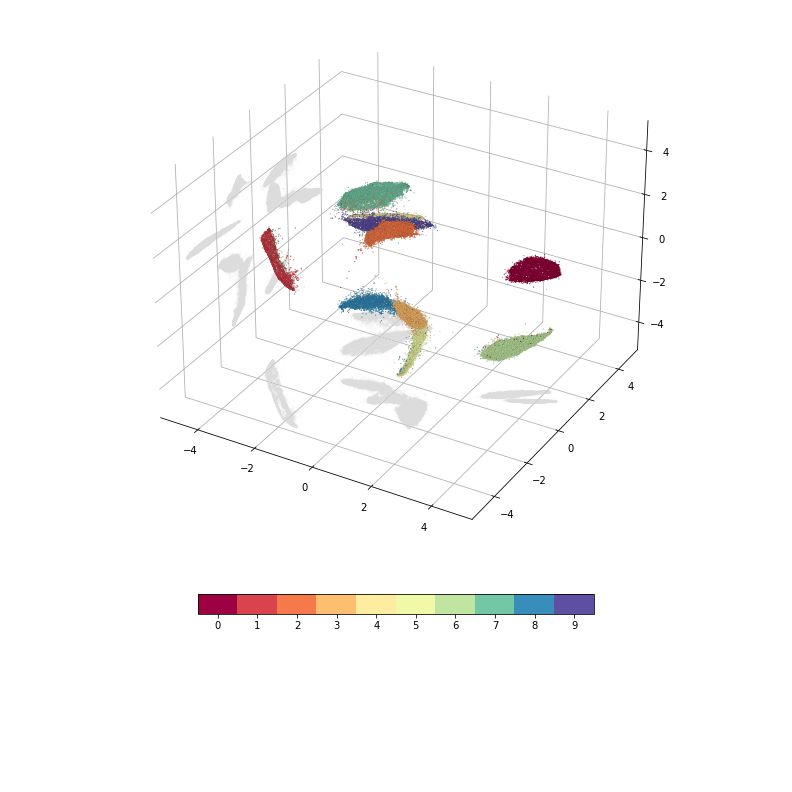#### Customizing embeddings

The `pymde.preserve_neighbors` function takes a few keyword arguments that can be used to customize the embedding. For example, you can impose a `pymde.Standardized` constraint: this causes the embedding to have uncorrelated columns, and prevents it from spreading out too much.

```embedding = pymde.preserve_neighbors(mnist.data, constraint=pymde.Standardized()).embed()
pymde.plot(embedding, color_by=mnist.attributes['digits'])
```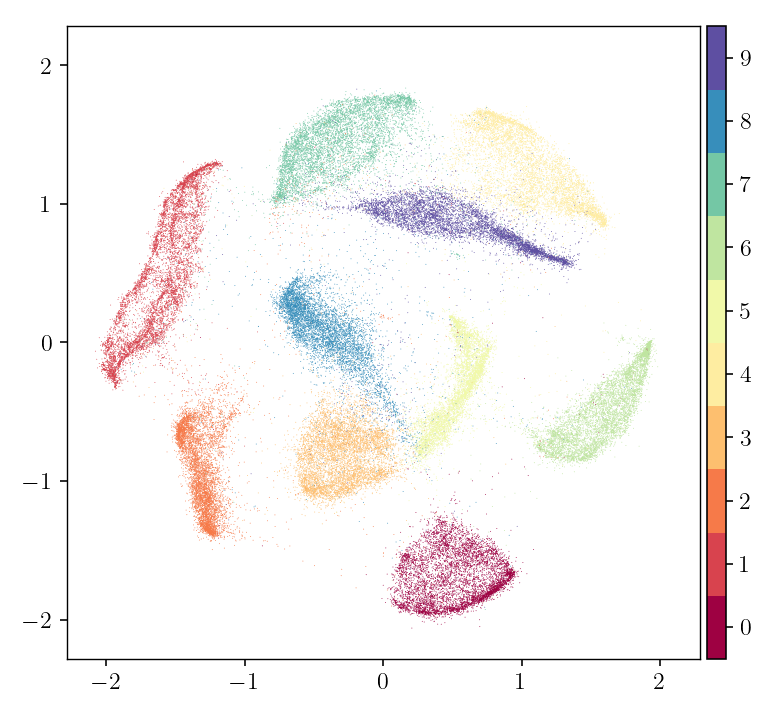To learn about the other keyword arguments, read the tutorial on MDE, then consult the API documentation.

For more in-depth examples of creating neighborhood-based visualizations, including 3D embeddings, see the MNIST and single-cell genomics example notebooks.

#### Accessing the underlying graph

You can access the graph underlying the MDE problem returned by `pymde.preserve_neighbors`, using the following code.

```edges = mde.edges
weights = mde.distortion_function.weights
```

The value `weights[i]` is the weight for the edge `edges[i]`.

### Preserving distances

Next, we’ll create an embedding that roughly preserves the global structure of some original data, by preserving some known original distances between some pairs of items. We will embed the nodes of an unweighted graph. For the original distance between two nodes, we’ll use the length of the shortest path connecting them.

The specific graph we’ll use is an academic coauthorship graph, from Google Scholar: the nodes are authors (with h-index at least 50), and two authors have an edge between them if either has listed the author as a coauthor.

```import pymde
import torch

device = 'cuda' if torch.cuda.is_available() else 'cpu'
embedding = mde.embed()
```

The `data` attribute of the `google_scholar` dataset is a `pymde.Graph` object, which encodes the coauthorship network. The `pymde.preserve_distances` function returns a `pymde.MDE` object, and calling the `embed` method computes the embedding.

Notice that we passed in a `device` to `pymde.preserve_distances`; this embedding approximately preserves over 80 million distances, so using a GPU can speed things up.

Next we plot the embedding, coloring each point by how many coauthors the author has in the network (normalized to be a percentile).

```pymde.plot(embedding, color_by=google_scholar.attributes['coauthors'])
```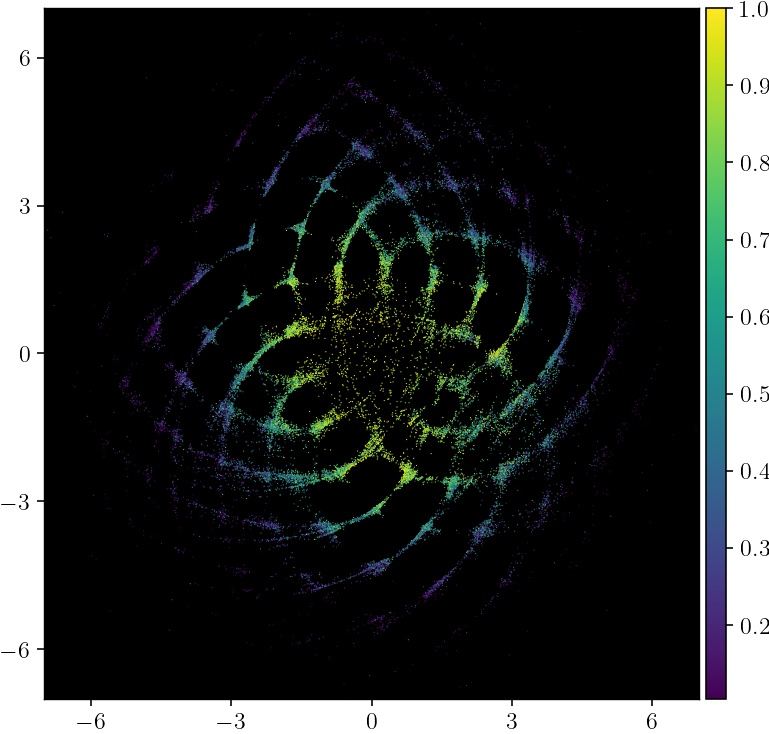The most collaborative authors are near the embedding, and less collaborative ones are on the fringe. It also turns out that the diameter of the embedding is close to the true diameter of the graph.

For a more in-depth study of this example, see the notebook on Google Scholar.

#### Customizing embeddings

The `pymde.preserve_distances` function takes a few keyword arguments that can be used to customize the embedding.

To learn about the keyword arguments, read the tutorial on MDE, then consult the API documentation.

#### Accessing the underlying graph

You can access the graph underlying the MDE problem returned by `pymde.preserve_distances`, using the following code.

```edges = mde.edges
distances = mde.distortion_function.deviations
```

The value `distances[i]` is the weight (which should be interpreted as a distance) for the edge `edges[i]`.

### Plotting

#### Scatter plots

The `pymde.plot` function can be used to plot embeddings with dimension at most 3. It takes an embedding as the argument, as well a number of optional keyword arguments. For example, to plot an embedding and color each point by some attribute, use:

```pymde.plot(embedding, color_by=attribute)
```

The `attribute` variable is a NumPy array of length `embedding.shape`, in which `attribute[k]` is a tag or numerical value associated with item `k`. For example, in the MNIST data, each entry in `attribute` is an `int` between `0` and `9` representing the digit depicted in the image; for single-cell data, each entry might be a string describing the type of cell. Typically the attribute is not used to create the embedding, so coloring by it is a sanity-check that the embedding has preserved prior knowledge about the original data.

This function can be configured with a number of keyword arguments, which can be seen in the `API documentation`.

#### Movies

The `pymde.MDE.play` method can be used to create an animated GIF of the embedding process. To create a GIF, first call `pymde.MDE.embed` with the `snapshot_every` keyword argument, then call `play`:

```mde.embed(snapshot_every=1)
mde.play(savepath='/path/to/file.gif')
```

The `snapshot_every=1` keyword argument instructs the `MDE` object to take a snapshot of the embedding during every iteration of the solution algorithm. The `play` method generates the GIF, and saves it to `savepath`.

This method can be configured with a number of keyword arguments, which can be seen in the `API documentation`.

## Generating feature vectors

The embeddings made via `pymde.preserve_neighbors` and `pymde.preserve_distances` can be used as feature vectors for supervised learning tasks. You can choose the dimension of the vectors by specifying the `embedding_dim` keyword argument, e.g.,

```embedding = pymde.preserve_neighbors(data, embedding_dim=50).embed()
```

## Classical embeddings

PyMDE provides a few implementations of classical embeddings, for convenience. To produce a PCA embedding of a data matrix, use the `pymde.pca` method, which returns an embedding:

```embedding = pymde.pca(data_matrix, embedding_dim)
```

To create a Laplacian embedding based on the nearest neighbors of each row in a data matrix or each node in a graph, use the `pymde.laplacian_embedding` method, which returns an MDE problem:

```mde = pymde.laplacian_embedding(data, embedding_dim, verbose=True)
embedding = mde.embed()
```

To create a spectral embedding based on a sequence of edges (a `torch.Tensor` of shape `(n_edges, 2)`) and weights, use `pymde.quadratic.spectral`. (These embeddings are called “quadratic embeddings” in the MDE monograph.)

## Drawing graphs

PyMDE can be used to draw graphs in 2 or 3 dimensions. Here is a very simple example that draws a cycle graph on 3 nodes.

```edges = torch.tensor([
[0, 1],
[0, 2],
[1, 2]
])
triangle = pymde.Graph.from_edges(edges)
triangle.draw()
```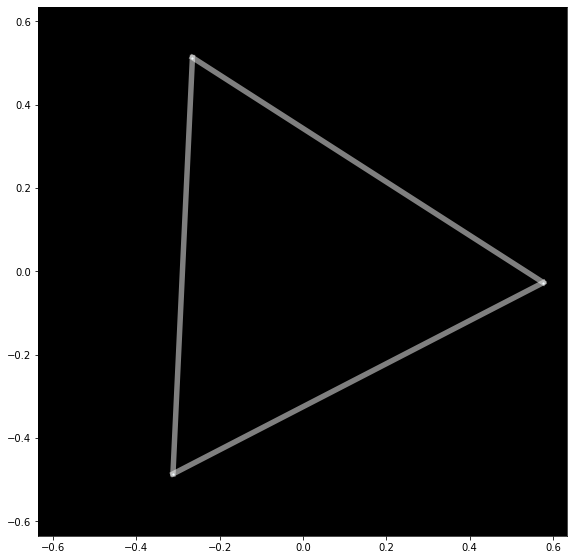Here is a more interesting example, which embeds a ternary tree. The tree is created using the NetworkX package.

```import networkx

ternary_tree = networkx.balanced_tree(3, 6)
embedding = graph.draw()
```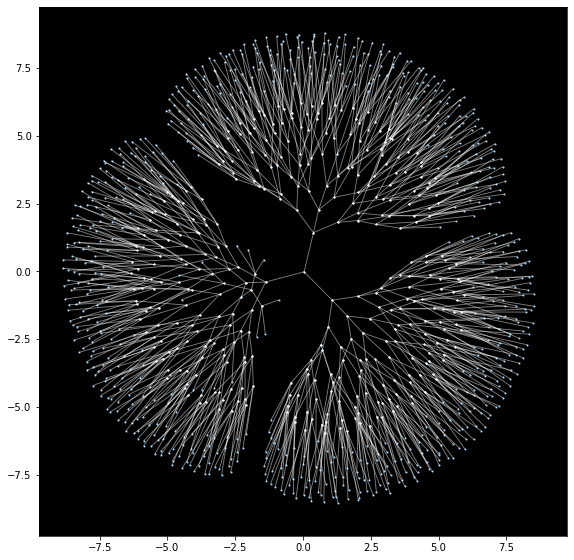On a standard CPU, it takes PyMDE just 2 seconds to compute this layout; for comparison, it takes NetworkX 30 seconds to compute a similar layout.

You can embed into 3 dimensions by passing `embedding_dim=3` to the `draw` method.

For more in-depth examples, see the notebook on drawing graphs, and the API documentation of `pymde.Graph`.

## Using a GPU

If you have a CUDA-enabled GPU, you can use it to speed up the optimization routine which computes the embedding.

The functions `pymde.preserve_neighbors` and `pymde.preserve_distances`, as well as the method `Graph.draw`, all take a keyword argument, called `device`, which controls whether or not a GPU is used. Pass `device='cuda'` to use your GPU. (PyMDE computes embeddings on CPU by default.)

For example, the below code shows how to create a neighbor-preserving embedding of MNIST using a GPU.

```import pymde

mnist = pymde.datasets.MNIST()
mde = pymde.preserve_neighbors(mnist.data, device='cuda', verbose=True)
embedding = mde.embed(verbose=True)
```

On an NVIDIA GeForce GTX 1070, the `embed` method took just 5 seconds.

## Reproducibility

PyMDE’s optimization algorithm does not rely on randomness. However, some functions, such as `pymde.preserve_neighbors`, may use random sampling when generating the edges for an MDE problem. This means that if you call `pymde.preserve_neighbors` multiple times on the same data set, you might obtain slightly different MDE problems and therefore slightly different embeddings. To prevent this from happening, you can set random seed state via the `pymde.seed` function.

For example, the following code block will always create the same embedding.

```import pymde

mnist = pymde.datasets.MNIST()

pymde.seed(0)
mde = pymde.preserve_neighbors(mnist.data, verbose=True)
embedding = mde.embed(verbose=True)
```